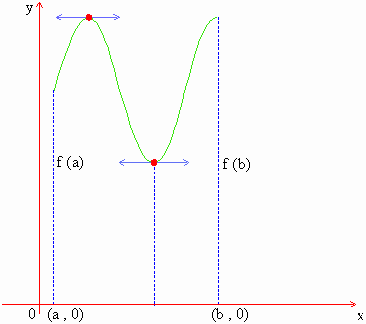Home MonkeyNotes Printable Notes Digital Library Study Guides Study Smart Parents Tips College Planning Test Prep Fun Zone Help / FAQ How to Cite New Title Request

5. 8 The Mean-value Theorem

For the theoretical purpose, the mean-value theorem is one of the most useful tools. The special and easy case of mean value theorem is the Rolle's theorem, which will serve as a lemma for our main results.

Rolles Theorem : If f is continuous on [a, b] and differentiable in (a, b) and if, further f (b) = f (a), then their exists at least one point x = c in (a, b) such that f '(c ) = 0Your browser does not support the IFRAME tag.

In this theorem, y = f (x) is continuous on [a, b] and differentiable in (a, b). Also f (b) = f (a). Hence the curve y = f (x) can be drawn as in the adjoining figure. The result f  ( c ) = 0 means the tangent at x = c is parallel to x - axis. Hence y = f (x) is continuous on [a,b], differentiable in (a, b) and f (b) = f (a), then there exists at least one point on this curve at which the tangent is parallel to x - axis.

Index

5.1 Tangent And Normal Lines
5.2 Angle Between Two Curves
5.3 Interpretation Of The Sign Of The Derivative
5.4 Locality Increasing Or Decreasing Functions 5.5 Critical Points
5.6 Turning Points
5.7 Extreme Value Theorem
5.8 The Mean-value Theorem
5.9 First Derivative Test For Local Extrema
5.10 Second Derivative Test For Local Extrema
5.11 Stationary Points
5.12 Concavity And Points Of Inflection
5.13 Rate Measure (distance, Velocity And Acceleration)
5.14 Related Rates
5.15 Differentials : Errors And Approximation